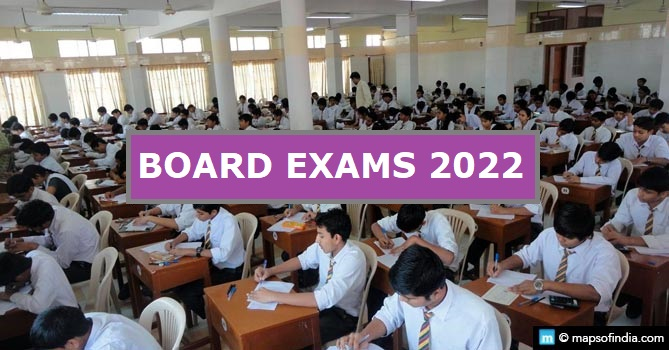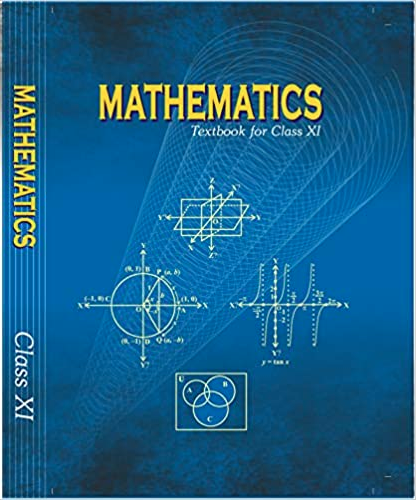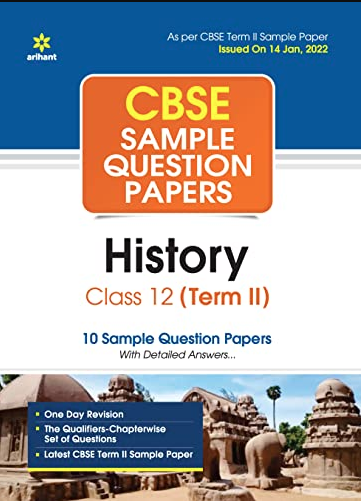# Colleges in Bangalore University

Founded in 1964, the Bangalore University is renowned for providing degree in several fields namely Engineering, Education, Science, Law, Medical, Arts, Commerce and Management and more.

There are nearly 473 affiliated colleges, 3 constituent college, 41 post-graduate departments and Directorates of Higher learning under Bangalore University.

Here is a list of the several Bangalore Universties Colleges.

## Medical Colleges Under Bangalore University

 Management Institutes Under Bangalore University

 Law College Under Bangalore University

 IT Undergraduate Colleges Under Bangalore University

Last Updated on : November 13, 2017Class 12 Physics Exam Today’s Term 2 Question Paper & Answer KeyThe CBSE Class 12 term 2 Physics exam concluded today, with students coming out from centres and stating that it was moderately balanced. It included concept-based questions. Some students said… Read More...Chapter 5 - Complex Numbers and Quadratic Equations Questions and Answers: NCERT Solutions for Class 11 MathsExercise 5.1 1. Express the given complex number in the form a+ib:(5i)(−35i)a+ib:(5i)(−35i) And evaluate Ans: Evaluate the complex number (5i)(−35i)=−5×35×i×i(5i)(−35i)=−5×35×i×i (5i)(−35i)=−3i2⋯[i2=−1](5i)(−35i)=−3i2⋯[i2=−1] (5i)(−35i)=3(5i)(−35i)=3 2. Express the given complex number in the… Read More...Chapter 4 - Principle of Mathematical Questions and Answers: NCERT Solutions for Class 11 Maths( Exercise 4.1) 1. Prove that following by using the principle of mathematical induction for all n∈Nn∈N: 1+3+32+.....+3n-1=(3n-1)21+3+32+.....+3n-1=(3n-1)2 Ans: Let us denote the given equality by P(n),P(n), i.e., P(n):1+3+32+.....+3n-1=(3n-1)2P(n):1+3+32+.....+3n-1=(3n-1)2 For… Read More...Mention any two places related to the Revolt of 1857.Mention any two places related to the Revolt of 1857. Answer. Mention any two places related to the Revolt of 1857. Jhansi, Delhi, Calcutta, Awadh, Kanpur, Meerut Read More...